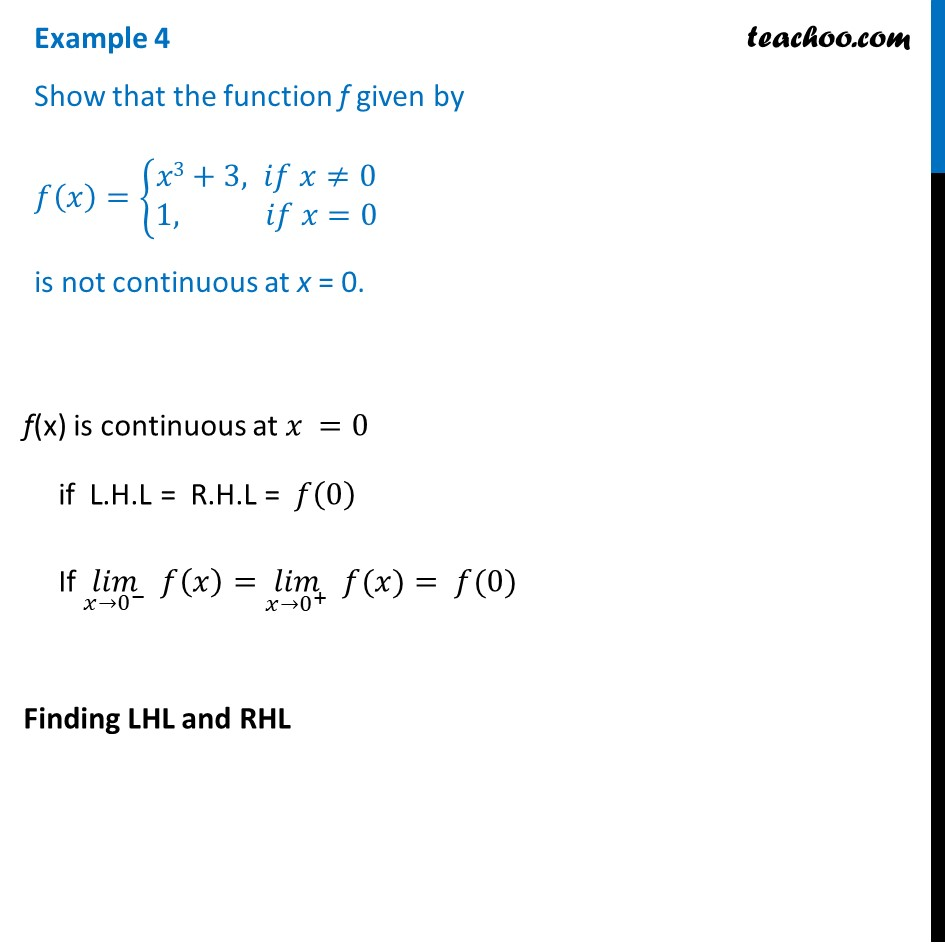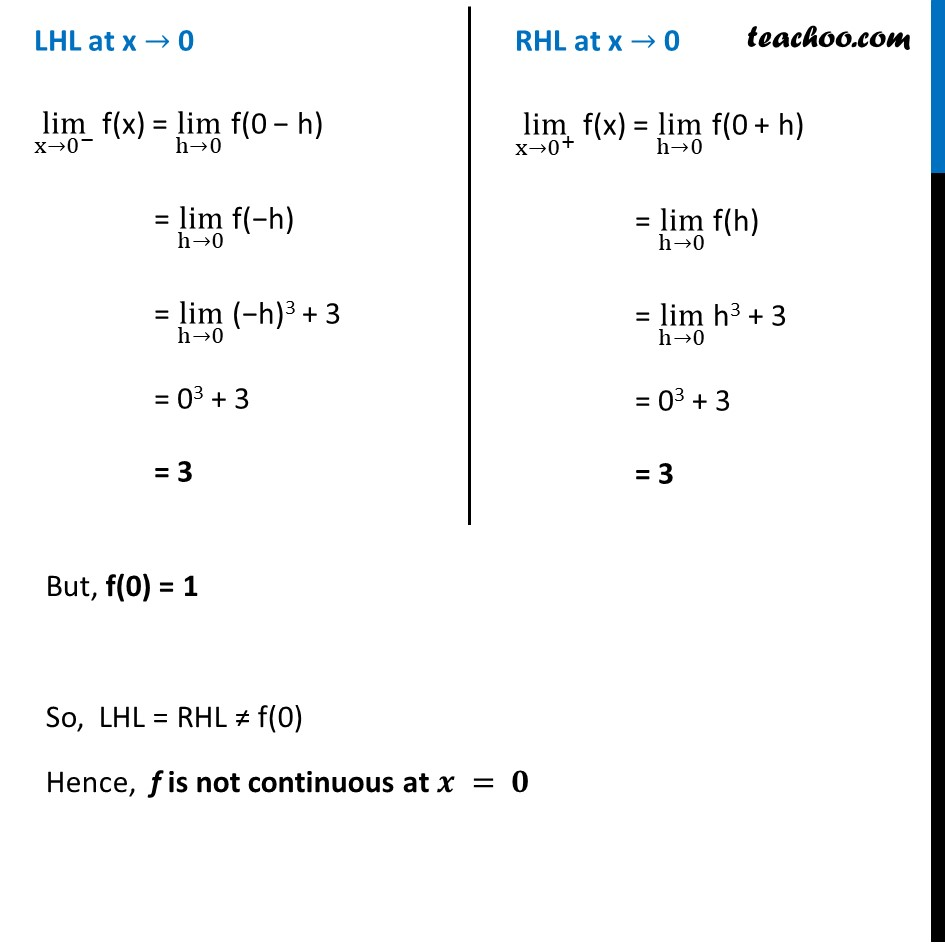Check Full Chapter Explained - Continuity and Differentiability - Continuity and Differentiability Class 121. Chapter 5 Class 12 Continuity and Differentiability
2. Serial order wise
3. Examples

Transcript

Example 4 Show that the function f given by 𝑓(𝑥)={█(𝑥3+3, 𝑖𝑓 𝑥≠0@1, 𝑖𝑓 𝑥=0)┤ is not continuous at x = 0. f(x) is continuous at 𝑥 =0 if L.H.L = R.H.L = 𝑓(0) If (𝑙𝑖𝑚)┬(𝑥→0^− ) 𝑓(𝑥)=(𝑙𝑖𝑚)┬(𝑥→0^+ ) " " 𝑓(𝑥)= 𝑓(0) Finding LHL and RHL LHL at x → 0 lim┬(x→0^− ) f(x) = lim┬(h→0) f(0 − h) = lim┬(h→0) f(−h) = lim┬(h→0) (−h)3 + 3 = 03 + 3 = 3 RHL at x → 0 lim┬(x→0^+ ) f(x) = lim┬(h→0) f(0 + h) = lim┬(h→0) f(h) = lim┬(h→0) h3 + 3 = 03 + 3 = 3 RHL at x → 0 lim┬(x→0^+ ) f(x) = lim┬(h→0) f(0 + h) = lim┬(h→0) f(h) = lim┬(h→0) h3 + 3 = 03 + 3 = 3 But, f(0) = 1 So, LHL = RHL ≠ f(0) Hence, f is not continuous at 𝒙 = 𝟎

Examples

About the AuthorDavneet Singh
Davneet Singh is a graduate from Indian Institute of Technology, Kanpur. He has been teaching from the past 10 years. He provides courses for Maths and Science at Teachoo.